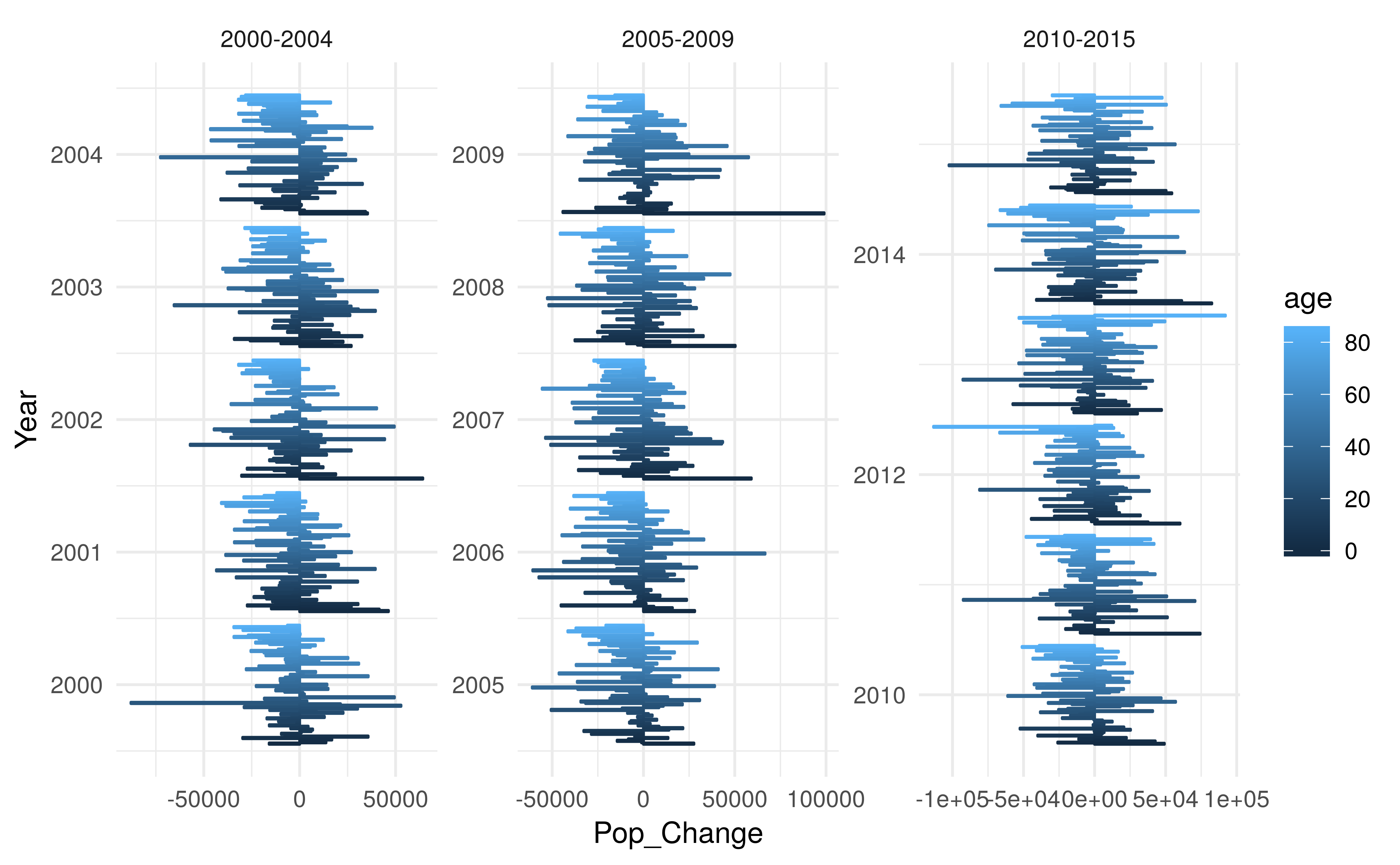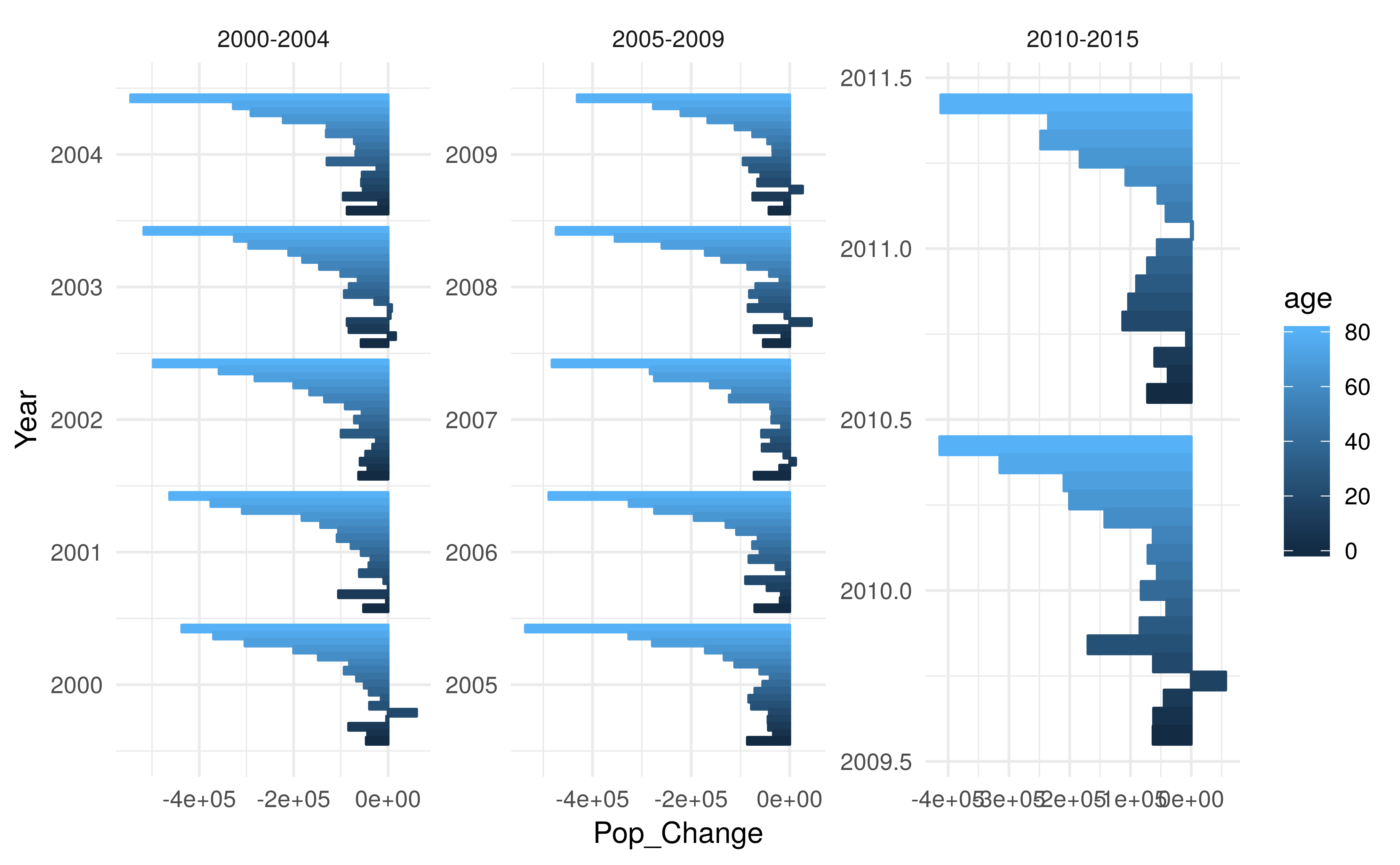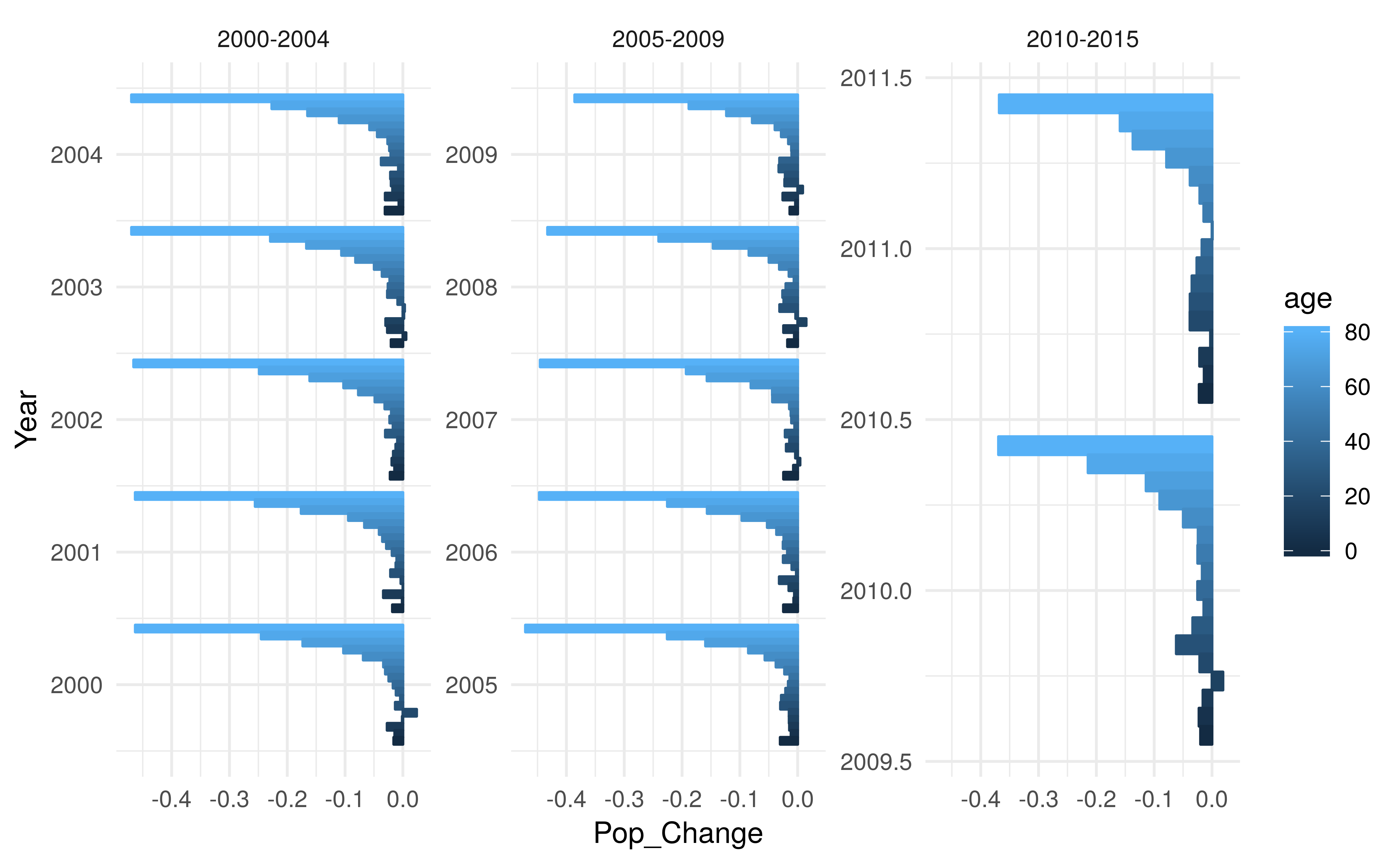# Estimating Population changes in the UK born population using the ONS, and LFS data

## Outline

• Attempt to use estimated population of the UK born to estimate population changes, capturing both mortality and emmigration.
• Split by single year of age, and by 5 year age group
• Compare results
• Restricted analysis to those aged under 85 as high levels of noise above this, and those aged 90+ are represented in a single category making this analysis infeasible.

## Implementation

library(tbinenglanddataclean)
library(tidyverse)
## data paths - run the making data vignette to get this data locally
birth_path = "../data/tb_data/tbinenglanddataclean/england_births.rds"
demo_path = "../data/tb_data/tbinenglanddataclean/E_ons_lfs_2000_2016.rds"

## read in data
demographics <- readRDS(demo_path)
## munge births
births <- births %>%
rename(Year = year, Births = births, Data = data) %>%
mutate(Age = as.character(0)) %>%
select(-Data)
## clean single year
demographics_single_year <- demographics %>%
ungroup %>%
select(-Age group, -Age group (condensed)) %>%
mutate(Age = as.character(Age)) %>%
filter(CoB %in% "UK born") %>%
select(-CoB)
## summarise by age group
demographics_5yr <- demographics %>%
ungroup %>%
group_by(Year, Age group, CoB) %>%
summarise(Population = sum(Population), Age = Age) %>%
ungroup %>%
mutate(Age = as.character(Age)) %>%
filter(CoB %in% "UK born") %>%
select(-CoB)
estimate_pop_changes <- function(demographics, births) {
##Join dataframes
demographics <- demographics %>%
full_join(births, by = c("Year", "Age")) %>%
mutate(Births = Births %>%
replace(is.na(Births), 0)) %>%
filter(Year >= 2000)

## set up age baseline and estimate population changes
demographics <- demographics %>%
mutate(age = Age %>%
replace(Age %in% "90+", "90") %>%
as.numeric) %>%
mutate(age_baseline = age - (Year - 2000)) %>%
group_by(age_baseline) %>%
mutate(Pop_Change = (lead(Population) - Population)) %>%
arrange(age_baseline)

return(demographics)
}
## Estimate for grouped data
demographics_5yr <- estimate_pop_changes(demographics_5yr, births)

## Estimate for single year data
demographics_single_year <- estimate_pop_changes(demographics_single_year, births)
visualise_pop_changes <- function(demographics, rate = FALSE, scales = "free") {

if (rate) {
demographics <- mutate(demographics, Pop_Change = Pop_Change/Population)
}
##  basic plot to visualise
demographics %>%
mutate(Years = case_when(Year < 2005 ~ "2000-2004",
Year >= 2005 & Year < 2010 ~ "2005-2009",
Year >= 2010 & Year < 2016 ~ "2010-2015")) %>%
na.omit() %>%
filter(age < 85) %>%
mutate(Year = as.integer(Year)) %>%
ggplot(aes(x = Year, y = Pop_Change, colour = age, fill = age,
group = age)) +
geom_bar(stat = "identity", position = "dodge") +
coord_flip() +
facet_wrap(~Years, scales = scales) +
theme_minimal()
}

## Results

### Single year of age estimates

Single year of age estimates are to noisy to be useful. This is likely due to varing sampling error between years for the labour force survey. This indicates that the data should only be used with caution to estimate the UK born population. There is little evidence of systematic bias.

visualise_pop_changes(demographics_single_year)### 5 year age grouped estimates

• Grouping by 5 year age categories reduced the amount of noise we observe in estimated population changes
• However it also reduces the number of years with viable data from 2000-2015, to 2000-2011
• Evidence of exponential increase in deaths
visualise_pop_changes(demographics_5yr)• Investigating rates we observe some evidence of decreasing mortality over time.
• There is also evidence of higher emmigration/mortality in young adults
• There is some evidence of an increasing population in those aged 15-19, this may be noise that has not been removed through aggregation. However it may also be due to individuals returning to the UK for education/work once they have reached 18.
visualise_pop_changes(demographics_5yr, rate = TRUE, scales = "free_y")## Conclusions

• Single year of age estimates are too noisy to be used to estimate population changes
• When grouped by 5 year age groups more reliable estimates can be made
• The inclusion of those emigrating and possibly returning adds complexity that may be difficult to account for in a modelled population
• Grouping the data reduces the number of available years of data
• It may be more sensible to use mortality estimates for the total English population (as published by ONS) and assume that mortality rates are equivalent in the UK born.